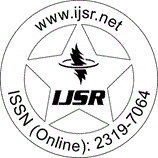International Journal of Science and Research (IJSR)
Call for Papers | Fully Refereed | Open Access | Double Blind Peer Reviewed

Research Paper | Mathematics | Nigeria | Volume 7 Issue 8, August 2018

# Methods for Determining Fractal Dimensions

Maurice Nnamdi ANNORZIE

Abstract: In this paper, A is considered to be a non-empty compact subset of a metric space X. For each small positive number, the minimum number of closed balls of radius, needed to cover A is denoted by N (A,). The objective is to obtain the fractal dimension of A, denoted by D (A), which is the limit as tends to zero of the ratio if this limit exists. Three main methods are discussed with relevant geometrical and theoretical ideas leading to determination of fractal dimension of the given set. The methods showed that the fractal dimension, D (A), exists for all compact subset of n-dimensional Euclidean space, , where D (A) is equal to both the Hausdorff-Besicovitch dimension, D_H and the Packing dimension, D_P due to self-similarity property of fractals.

Keywords: Fractals, Fractal Dimension, Hausdoff-Besicovitch Dimension, Packing Dimension and Self-similarity

Edition: Volume 7 Issue 8, August 2018,

Pages: 1653 - 1656